# Algebra II : Radicals as Exponents

## Example Questions

1 2 3 5 Next →

### Example Question #92 : Understanding Radicals

Solve: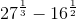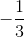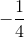Explanation:

The denominator of the fractional exponent represents the index of the radical.

Rewrite the expression in radical form.  A radical with an index of 2 is simply the square root of a number.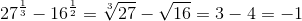The answer is:### Example Question #93 : Understanding Radicals

What is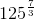equivalent to?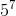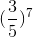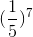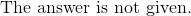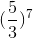Explanation:

The denominator represents the index of the square root.  The numerator is the  power that the quantity is raised to.

Rewrite the expression as a radical.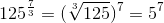The answer is:1 2 3 5 Next →

### All Algebra II Resources